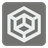Count Inversions
Elementary+
English EL ES FR IT JA RU UK

In computer science and discrete mathematics, an inversion is a pair of places in a sequence where the elements in these places are out of their natural order. So, if we use ascending order for a group of numbers, then an inversion is when larger numbers appear before lower number in a sequence.

Check out this example sequence: (1, 2, 5, 3, 4, 7, 6) and we can see here three inversions
- 5 and 3;
- 5 and 4;
- 7 and 6.

You are given a sequence of unique numbers and you should count the number of inversions in this sequence.

Input: A sequence as a tuple of integers.

Output: The inversion number as an integer.

Example:

```count_inversion((1, 2, 5, 3, 4, 7, 6)) == 3
count_inversion((0, 1, 2, 3)) == 0
```

How it is used: In this mission you will get to experience the wonder of nested loops, that is of course, if you don't use advanced algorithms.

Precondition: 2 < len(sequence) < 200
len(sequence) == len(set(sequence))
all(-100 < x < 100 for x in sequence)